GFG App
Open AppBrowser
Continue

# Perimeter of a Parallelogram

The perimeter of a parallelogram is the sum of the length of its boundaries/sides. A parallelogram is a type of quadrilateral with four equal sides with opposite sides equal. Its sides do not intersect each other. There are two diagonals of a parallelogram that intersect each other at the center. A diagonal divides the parallelogram into two equal parts or triangles. Following are the properties of parallelogram:

1. A parallelogram has four sides.
2. Opposite sides of parallelograms are equal and parallel.
3. Opposite angles of parallelograms are equal.
4. Diagonals of parallelograms intersect each other.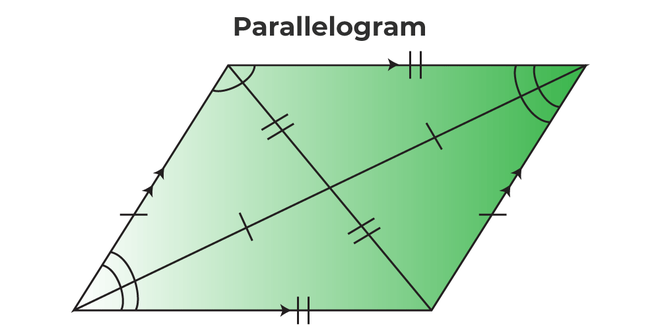## What is the Perimeter of a Parallelogram?

The perimeter of a parallelogram is the length of the outline or its boundaries, and the sum of all the sides of a parallelogram is the perimeter of the parallelogram. However, not every time the length of all the sides will be given to us, sometimes some other information regarding a parallelogram is given. Therefore, there are different formulas for the perimeter of a parallelogram. Let’s understand the different formulas for the perimeter of a parallelogram.

## Perimeter of Parallelogram Formula

The perimeter of the parallelogram is the sum of the length of all the sides. Perimeter refers to the closed boundary of any geometrical object. For a quadrilateral, perimeter refers to the sum of the length of the four sides. So, the perimeter of a parallelogram will be the sum of its four sides. The perimeter of a parallelogram can be calculated using three cases. The three cases are:

• When the adjacent sides of the parallelogram are known: The formula for the perimeter when sides are known as P = 2(a + b) units, where a and b are the sides of the parallelogram.
• When one side and the lengths of the diagonals are known: The formula for the perimeter when one side and the lengths of diagonals are known, P = 2a + √(2x2 + 2y2 – 4a2), where x and y are the lengths of diagonals and a is the length of the side.
• When any angle, base, and height are known: The formula for the perimeter when one side and height along with one of the angles is given, P = 2a + 2h/sinθ, where a is the side of the parallelogram, h is the height of the parallelogram, θ is the angle of the parallelogram.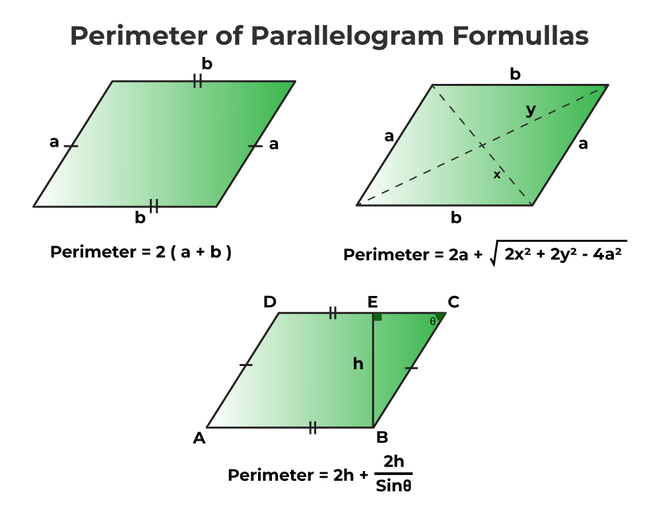Now that the formulas of all three cases are known let’s derive the formulas of the perimeter of a parallelogram for all three cases,

## Perimeter of Parallelogram Formula with Sides

The perimeter of the parallelogram formula with sides is the formula to calculate the perimeter. Below is the derivation for the perimeter of the parallelogram. Let’s say the sides of the parallelogram are “a” and “b”.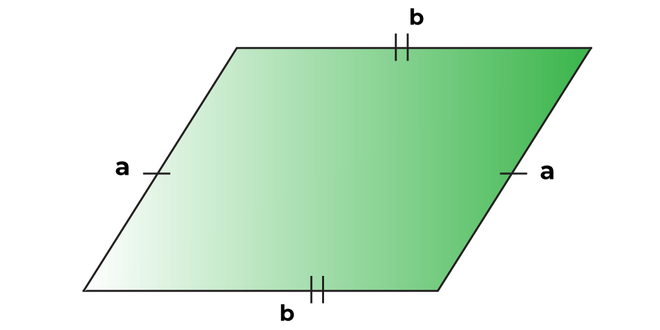Perimeter = side 1 + side 2 + side 3 + side 4

Side 1 is also known as the base of a parallelogram.

Side 1 = a

Side 2 = b

The opposite sides of a parallelogram are equal.

Side 1 = side 3

Side 2 = side 4

Side 3 = a

Side 4 = b

Perimeter = a + b + a + b

Perimeter = 2 (a + b)

Hence, the perimeter of a parallelogram is twice the sum of its two adjacent sides.

## Perimeter of Parallelogram Formula with One Side and Diagonals

The perimeter of the parallelogram formula when one side and the length of both diagonals are given is derived below. Let’s say the length of the diagonals are “x” and “y” and the length of the side is “a”, from the law of cosines, the cosine formula is applied: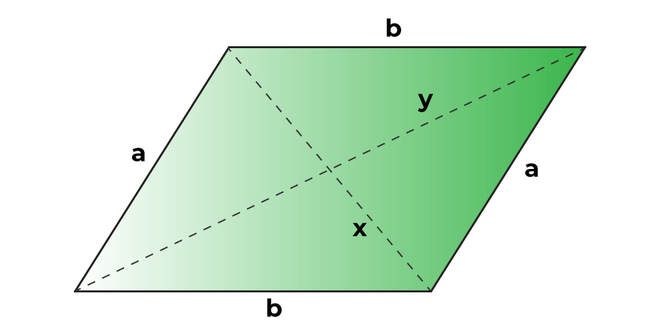In △ABD:

x2 = a2 + b2 -2ab cos∠BAD

y2 = a2 + b2 -2ab cos∠ADC

x2 + y2 = 2 (a2 + b2) – 2ab (cos∠BAD + cos∠ADC)

Now since according to the properties of parallelogram, the adjacent angles of a parallelogram are supplementary, therefore, ∠BAD + ∠ADC = 180.

Substituting the angle,

x2 + y2 = 2 (a2 + b2) – 2ab (-cos∠ADC + cos∠ADC)

x2 + y2 = 2 (a2 + b2) – 2ab (0)

x2 + y2 = 2 (a2 + b2)

Now finding the value of the b side from the equation formulated above:

b = √[(x2 + y2 – 2a2)/2]

Now that both sides of the parallelogram are known, using the formula for the perimeter of a parallelogram with sides,

P = 2(a + b)

P = 2a + 2(√[(x2 + y2 – 2a2)/2])

P = 2a + √(2x2 + 2y2 – 4a2)

## Perimeter of Parallelogram with Base, Height, and Angle

In order to find the perimeter of a parallelogram with base, height, and angle, let’s assume the base of the parallelogram is “b”, the height of the parallelogram is “h”, and the angle of the parallelogram is “θ”.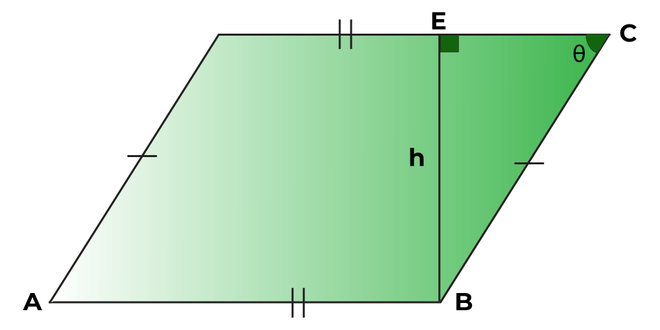Applying sin function:

sin θ = h/b

b = h/sin θ

Now, the length of the side “b” is known to us in terms of angle. Substituting the value of “b” in the formula:

P = 2(a + b)

P = 2a + 2h/sin θ

Note: θ can be the angle of any vertex of the parallelogram and the formula will remain the same.

## Area and Perimeter of Parallelogram

We can find the relation between the area and perimeter of a parallelogram because both the formulas contain sides of the parallelogram, the formula for the area of parallelogram and perimeter of a parallelogram is:

Area of parallelogram = A = b × h square units ⇢ (1)

Perimeter of a parallelogram = P = 2a + 2b units ⇢ (2)

Finding the value of b from equation (2)

P/2 = a + b

b = P/2 – a

Substituting the value of “b” obtained in equation (1)

A = (P/2 – a) h square units

## How to Find Perimeter of Parallelogram?

In order to find the perimeter of a parallelogram, the formulas must be known to us. The perimeter of a parallelogram is the sum of all four sides of the parallelogram. However, not every time all the sides are provided. In some cases, only one side and diagonals are given. In some case, height, angle, and a side is given. We have discussed the different formulas required for the different cases. Following are the steps that should be taken in order to find the perimeter of parallelogram:

• Note down the values given in the question.
• Based on the values given, apply the formula for the perimeter of parallelogram accordingly:

## Solved Examples on Perimeter of Parallelogram

Example 1: Find the perimeter of a parallelogram with side length = 14m, base = 10m.

Solution

The perimeter of parallelogram is given by:

2(a + b)

Where, a and b are its two adjacent sides

Perimeter = 2 (14 + 10)

Perimeter = 2 (24)

Perimeter = 48m

Example 2: Find the perimeter of a parallelogram whose base is 5cm, and the side length is 6cm.

Solution

The perimeter of a parallelogram is given by:

2(a + b)

Where, a is the base and b is its adjacent side

Perimeter = 2 (5 + 6)

Perimeter = 2 (11)

Perimeter = 22cm

Example 3: What is the perimeter of a parallelogram with a side length of 8 in, and diagonals are 12in and 10in?

Solution

The formula for the perimeter when one side and the lengths of diagonals are known,

P = 2a + √(2x2 + 2y2 – 4a2)

P = 2 × 8 + √(2(12)2 + 2(10)2 – 4(8)2)

P = 16 + √(288 + 200 – 4(64))

P = 31.23 in.

Example 4: What is the perimeter of a parallelogram when the height is 20cm, the vertex angle is 45°, and one of the sides is 12cm?

Solution

The perimeter of a parallelogram is given by:

P = 2a + 2h/sinθ

P = 2 × 12 + 2 × 20/sin45

P = 24 + 40 × 2

P = 24 + 80

P = 104 cm

Example 5: The perimeter of a parallelogram is 100cm, and one of the sides of the parallelogram is 32cm; find the length of the other side.

Solution

The perimeter of a parallelogram is given by:

P = 2(a + b)

Where a and b are its two adjacent sides

Given: P = 100cm, a = 32cm

100 = 2 (32 + b)

50 = 32 + b

b = 18cm

The length of the other side of the parallelogram is 18cm.

## FAQs on Perimeter of Parallelogram

Question 1: What is the formula for the perimeter of a parallelogram?

The formula for the perimeter of a parallelogram is given:

P = 2(a + b)

Where a and b are the adjacent sides of the parallelogram.

Question 2: How to find the perimeter of a parallelogram with a missing side?

When one side of the parallelogram is missing, the perimeter of the parallelogram can be found in either the length of the diagonals given or if the height along with the vertex angle is given.

Perimeter of a parallelogram with diagonals = 2a + √(2x2 + 2y2 – 4a2).

Perimeter of a parallelogram with height and vertex angle = 2a + 2h/sin θ.

Question 3: What is the perimeter of a parallelogram using base and height?

Solution:

We can find the perimeter of a parallelogram using the base and height when, along with the base and height, the vertex angle is also given. The formula for the perimeter of a parallelogram is,

P = 2a + 2h/sin θ

Where,

• a = side length
• h = height of the parallelogram
• θ = vertex angle

Question 4: What is the area of a parallelogram?

The area of the parallelogram is the region covered by the parallelogram in 2-D space. The formula for the area of a parallelogram is given as,

A = b × h square units

Where,

A = Area of the parallelogram

b = Base of the parallelogram

h = Height of the parallelogram

My Personal Notes arrow_drop_up